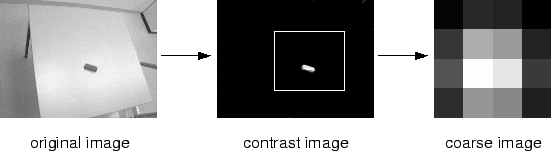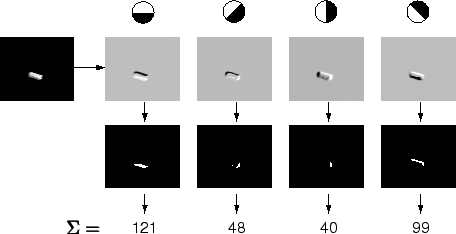Next: 6.2.4 Tuning curves Up: 6.2 Methods Previous: 6.2.2 Data collection

## 6.2.3 Image processing

The image processing extracts information on the position and on the orientation of the brick (section 6.1.2). The processing consists of several steps:

First, gray-scale images were produced using a contrast mechanism to enhance red (R - (G + B)/2, using the RGB-color code). In these images, a rectangle was determined that enclosed the brick in all images (figure 6.4). In the following, only the image region within this rectangle was further processed.

The brick appears almost as a white spot within the contrast image. Thus, a coarse grained version of the image provides a redundant code for the brick's position (figure 6.4). This population code was gained by using a grid of 4×4 `neurons' with Gaussian receptive fields. Their centers covered the image uniformly, and the Gaussian width was equal to the distance between two neighboring centers. The resulting 16 activation values were one part of a training pattern. Thanks to the blur in the coarse image, brick locations that were close to each other were also close in the space of the 16 activation values (compare to section 6.1.2).To obtain a population code for the orientation, the contrast image was first blurred. Then, four compass filters enhanced the edges in four different directions (figure 6.5). To the four resulting images, a threshold function was applied (figure 6.5). The remaining pixels in each image were counted to give a value for the distribution of edges in a given direction (figure 6.5). The result is a histogram showing the edge-direction distribution in the contrast image. Such a histogram can uniquely encode the orientation of the brick at a given location. The four values of this histogram were the second part of a training pattern.Next: 6.2.4 Tuning curves Up: 6.2 Methods Previous: 6.2.2 Data collection
Heiko Hoffmann
2005-03-22